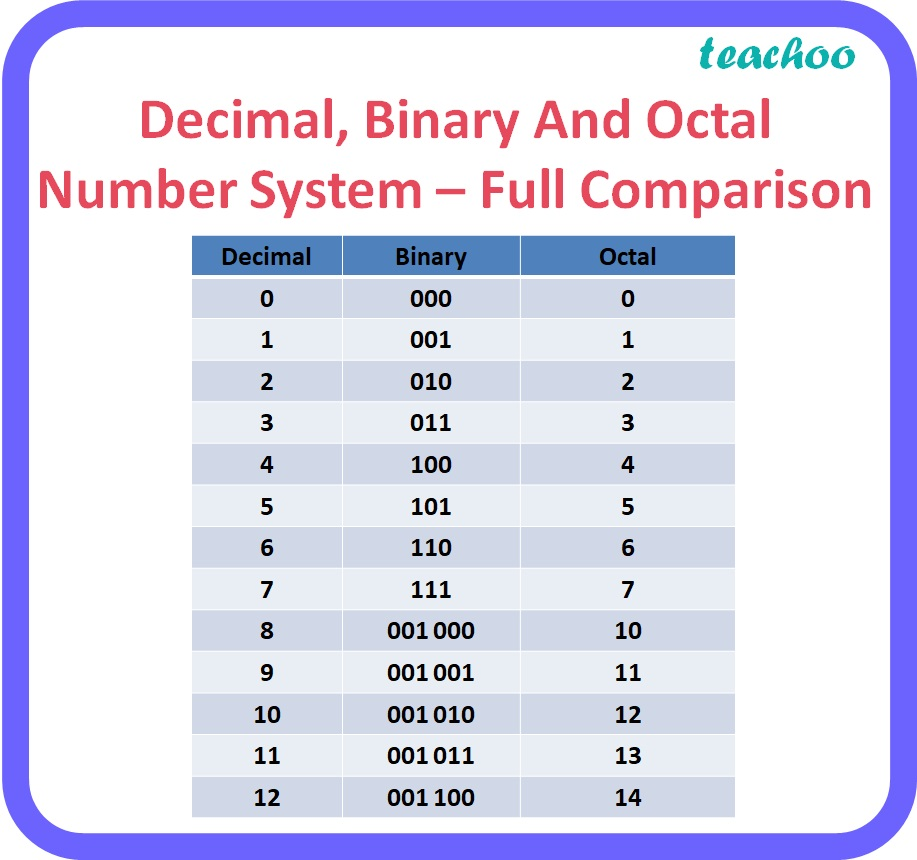3 Mark Questions

Computer Science - Class 11
Chapter 2 Class 11 - Encoding Schemes and Number System

## (b) 10010010

• 1011010
 00 1 011 010
 1 3 2

Therefore, 1011010 2 = 132 8

• 10010010

 0 10 010 010
 2 2 2

Therefore, 10010010 2 = 222 8

Explanation:

• To obtain the octal equivalent of a binary number, digits of binary number should be divided into groups of three digits starting from the rightmost digit for the integer part and starting from the leftmost digit for the fractional part.
• Convert each group of 3 digits to one octal digit as given in the table below.
• Note: 1 or 2 zeroes can be added before the leftmost digit or after the rightmost digit to make a complete group of 3 bits.Learn in your speed, with individual attention - Teachoo Maths 1-on-1 Class

### Transcript

Decimal, Binary And Octal Number System – Full Comparison